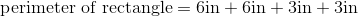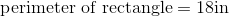# ISEE Lower Level Math : How to find the perimeter of a rectangle

## Example Questions

### Example Question #107 : Quadrilaterals

A rectangle measuresinches along its short side, andinches along its long side.  What is the perimeter of the rectangle?Explanation:

To find the perimeter of any object, simply add the length of each side together.  The best answer is: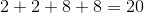### Example Question #108 : Quadrilaterals

A rectangle measuresinches along its short side, andinches along its long side.  What is the perimeter of the rectangle?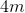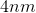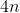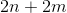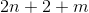Explanation:

To find the perimeter of any object, simply add the length of each side together.  The best answer is: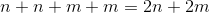### Example Question #109 : Quadrilaterals

Use the following to answer the question.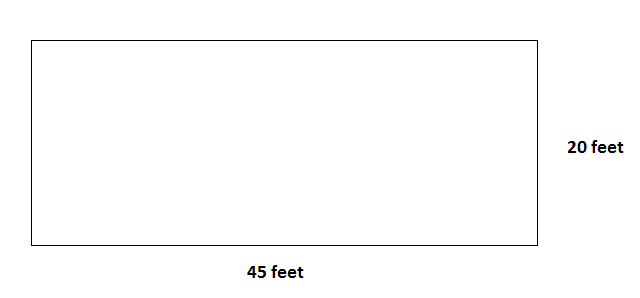You go to the community center to go swimming.  You see that the pool is a rectangular shape.  If the pool has a length of 45 feet and a width of 20 feet, what is the perimeter of the pool?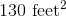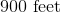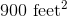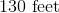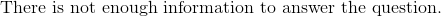Explanation:

To find the perimeter of a rectangle, we use the following formula: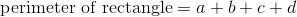where a, b, c, and d are the lengths of the sides of the rectangle.

Now, given the rectanglewe can see that the length is 45 feet.  We also see the width is 20 feet.  Now, because it is a rectangle, we know that opposite sides of the rectangle are equal.  In other words,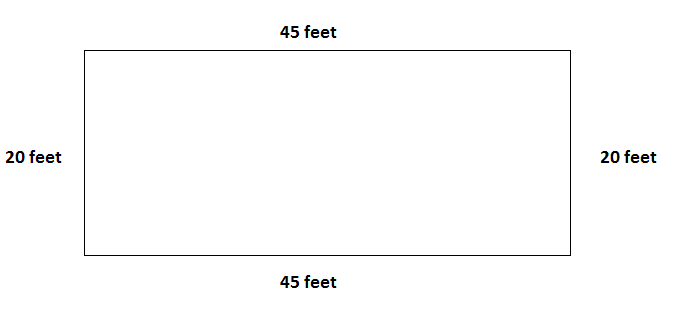So, now that we know the lengths of all the sides of the rectangle, we can substitute into the formula.  We get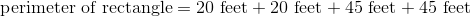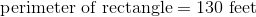### Example Question #110 : Quadrilaterals

Find the perimeter of a rectangle with a length of 10cm and a width that is half the length.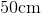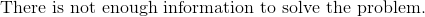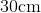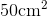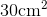Explanation:

To find the perimeter of a rectangle, we will use the following formula:where a, b, c, and d are the lengths of the sides of the rectangle.

Now, we know the length of the rectangle is 10cm.  We also know that because it is a rectangle, the opposite length is also 10cm.

We know the width of the rectangle is half the length.  Therefore, the width is 5cm.  We also know that because it is a rectangle, the opposite width is also 5cm.

Now, knowing all of this, we can substitute into the formula.  We get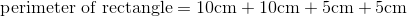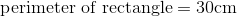### Example Question #31 : How To Find The Perimeter Of A Rectangle

Find the perimeter of a rectangle with a length of 14 inches and a width that is half the length.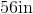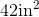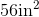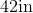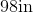Explanation:

To find the perimeter of a rectangle, we will use the following formula:where a, b, c, and d are the lengths of the sides of the rectangle.

Now, we know the length of the rectangle is 14 inches.  Because it is a rectangle, we know the opposite side is the same length.  Therefore, the opposite side is also 14 inches.  We also know the width is half the length.  Therefore, the width is 7 inches.  Because it is a rectangle, we know the opposite side is the same length.  Therefore, the opposite side is also 7 inches.  Knowing this, we can substitute into the formula.  We get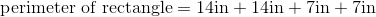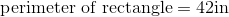### Example Question #32 : How To Find The Perimeter Of A Rectangle

Find the perimeter of a rectangle with a length of 10cm and a width that is half the length.Explanation:

To find the perimeter of a rectangle, we will use the following formula:where a, b, c, and d are the lengths of the sides of the rectangle.

Now, we know the base of the rectangle has a length of 10cm.  Because it is a rectangle, we know the opposite side is the same length.  Therefore, the opposite side is also 10cm.

We know the width is half the length.  Therefore, the width is 5cm.  Because it is a rectangle, we know the opposite side is the same length.  Therefore, the opposite side is also 5cm.

Knowing this, we can substitute into the formula.  We get### Example Question #33 : How To Find The Perimeter Of A Rectangle

Find the perimeter of a rectangle with a length of 10 inches and a width that is twice the length.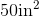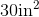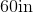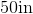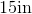Explanation:

To find the perimeter of a rectangle, we will use the following formula:where a, b, c, and d are the lengths of the sides of the rectangle.

Now, we know the length of the rectangle is 10 inches.  Because it is a rectangle, we know the opposite side is also 10 inches.  We also know the width is twice the length.  Therefore, the width is 20 inches.  Because it is a rectangle, we know the opposite side is also 20 inches.  Knowing all of this, we can substitute into the formula.  We get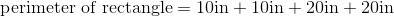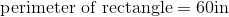### Example Question #34 : How To Find The Perimeter Of A Rectangle

Find the perimeter of a rectangle with a length of 7cm and a width of 4cm.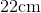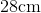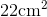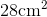Explanation:

To find the perimeter of a rectangle, we will use the following formula:where a, b, c, and are the lengths of the sides of the rectangle.

Now, we know the length of the rectangle is 7cm. Because it is a rectangle, we know the opposite side is also 7cm.  We also know the width of the rectangle iis 4cm.  Because it is a rectangle, the opposite side is also 4cm.  Knowing this, we can substitute into the formula.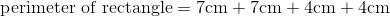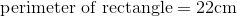### Example Question #35 : How To Find The Perimeter Of A Rectangle

Find the perimeter of a rectangle with a length of 7cm and a width of 3cm.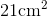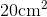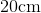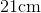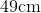Explanation:

To find the perimeter of a rectangle, we will use the following formula:where a, b, c, and d are the lengths of the sides of the rectangle.

Now, we know the length of the rectangle is 7cm.  Because it is a rectangle, we know the opposite side is also 7cm.  We know the width of the rectangle is 3cm.  Because it is a rectangle, we know the opposite side is also 3cm.  Knowing all of this, we can substitute into the formula.  We get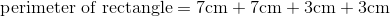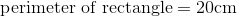### Example Question #36 : How To Find The Perimeter Of A Rectangle

Use the following figure to answer the question: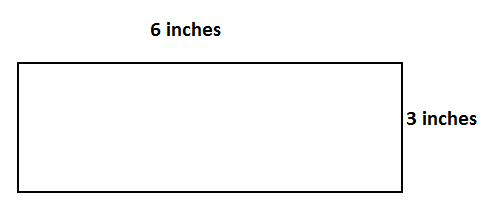Find the perimeter.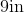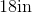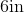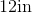Explanation:

To find the perimeter of a rectangle, we will use the following formula:where a, b, c, and d are the lengths of the sides of the rectangle.

Now, in the rectanglewe can see the length is 6 inches.  Because it is a rectangle, we know the opposite side is also 6 inches.

We can also see the width is 3 inches.  Because it is a rectangle, we know the opposite side is also 3 inches.

Knowing this, we can substitute into the formula.  We get